Lucy, Tereza, and Petra decided to compile the compulsory reading records together. It would take 30 days for Lucia alone, 36 for Tereza and 45 for Petra. How long will take make records if they will work together?

Result

t =  12 d

Solution:

t * (1/30+1/36+1/45) = 1

15t = 180

t = 12

Calculated by our simple equation calculator.

Leave us a comment of this math problem and its solution (i.e. if it is still somewhat unclear...):Be the first to comment!To solve this verbal math problem are needed these knowledge from mathematics:

Looking for help with calculating harmonic mean? Looking for a statistical calculator? Need help calculate sum, simplify or multiply fractions? Try our fraction calculator. Do you have a linear equation or system of equations and looking for its solution? Or do you have quadratic equation?

Next similar math problems:

1. Four painters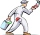The company sent four painters to paint the school. They should be done in 12 days. After three days, one painter got ill. How long will the remaining painters paint the school?
2. Trucks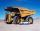Truck take you the some amount of clay in 30 hours. Second truck in 40 hours. Trucks carted the clay away some time together then the second truck broke down and the first one completed the job in five hours. What time worked together?
3. Digging companies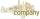Company A would dig a pit for 12 days, company B for 15 days, company C for 20 days and the company D for 24 days. Work began together companies C and D, but after three days joined them other two companies. How long took to dig a pit?
4. NovakNovak needed to dig up three of the same pit in the garden. The first pit dug father alone for 15 hours. His second dig son helped him and it did that in six hours. The third pit dug son himself. How long it took him?
5. Two pumps togetherThe first pump will fill the tank itself in 3 hours and the second one in 6 hours. How many hours will the tank be full if both pumps are worked at the same time?
6. Working togetherOne painter paint the nursery in 10 days, the second in 8 days. How many days will the nursery be painted if they work together?
7. Two typists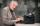There are two typists who are rewriting the material 814 pages. First can it handle rewrite yourself for 24 days; the second 12 days. First typist wrote material yourself 4 days rest rewrites yourself second typist. How many days will it take rewriting alt
8. Fifth of the numberThe fifth of the number is by 24 less than that number. What is the number?
9. Simple equation 6Solve equation with one variable: X/2+X/3+X/4=X+4
10. The tank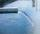The tank had 9 inflows to be filled in 21 days. After 9 days, 3 trips out. How many days did the remaining 6 tributaries fill the tank?
11. Bricklayers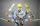When a bricklayer works himself to redeem the house in 8 days, the other bricklayer will be finished in 10 days. How long will it take to make 3 such houses together?
12. Hectoliters of waterThe pool has a total of 126 hectoliters of water. The first pump draws 2.1 liters of water per second. A second pump pumps 3.5 liters of water per second. How long will it take both pumps to drain four-fifths of the water at the same time?
13. Tonic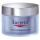One package tonic consume Mom 12 weeks and daughter for 14 weeks. 4 weeks they use the same cream together and then only daughter. How many weeks endured tonic to daughter then?
14. Equation with mixed fractions2 3/5 of 1430+? = 1900. How to do this problem
15. Three peopleThree people start doing a work at a same time. The first worked only 2 hours. The second ended 3 hours before the end. On an individual basis, it would take the first time to do the work 10 hours, second 12 hours and 15 hours third. How many hours did it.
16. Three monksThree medieval monks has task to copy 600 pages of the Bible. One rewrites in three days 1 page, second in 2 days 3 pages and a third in 4 days 2 sides. Calculate for how many days and what day the monks will have copied whole Bible when they begin Wednesd
17. Six yearsIn six years Jan will be twice as old as he was six years ago. How old is he?